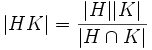# Hall satisfies permuting transfer condition

This article gives the statement, and possibly proof, of a subgroup property (i.e., Hall subgroup) satisfying a subgroup metaproperty (i.e., permuting transfer condition)
View all subgroup metaproperty satisfactions | View all subgroup metaproperty dissatisfactions |Get help on looking up metaproperty (dis)satisfactions for subgroup properties
Get more facts about Hall subgroup |Get facts that use property satisfaction of Hall subgroup | Get facts that use property satisfaction of Hall subgroup|Get more facts about permuting transfer condition

## Statement

### Statement with symbols

Suppose$H$ is a Hall subgroup of a finite group$G$. Suppose, further, that$K$ is a subgroup of$G$ such that$H$ and$K$ are permuting subgroups -- in other words,$HK = KH$. Then,$H \cap K$ is a Hall subgroup of$K$.

## Definitions used

### Hall subgroup

Further information: Hall subgroup

A subgroup$H$ of a finite group$G$ is termed a Hall subgroup if its order$|H|$ and its index$[G:H]$ are relatively prime.

## Facts used

1. Index is multiplicative
2. Lagrange's theorem
3. Product formula: This states that if$H$ and$K$ are subgroups of$G$, we have:$|HK| = \frac{|H||K|}{|H \cap K|}$.

## Proof

Given: A finite group$G$, a Hall subgroup$H$ of$G$, a subgroup$K$ of$G$ such that$HK = KH$.

To prove:$H \cap K$ is Hall in$K$.

Proof: Rearranging the product formula (fact (3)) yields:$\frac{|K|}{|H \cap K|} = \frac{|HK|}{|H|}$.

By Lagrange's theorem (fact (2)), and noting that$HK$ is a subgroup of$G$, we get:$[K:H \cap K] = [HK:H]$.

By fact (1), we have:$[HK:H][G:HK] = [G:H]$.

Thus, we get:$[K:H \cap K][G:HK] = [G:H]$.

In particular,$[K:H \cap K]$ divides$[G:H]$. By Lagrange's theorem, we have that$|H \cap K|$ divides$|H|$. Since$|H|$ and$[G:H]$ are relatively prime, we obtain that$[K:H \cap K]$ and$|H \cap K|$ are relatively prime. Thus,$H \cap K$ is a Hall subgroup of$K$.